CHAPTER III Applications of the Derivative (Draft- 2014 work in progress)
� 2014 M. Flashman.

III.A. ESTIMATIONS USING THE DERIVATIVE

III.A.2 NEWTON'S METHOD

Motivation: An apocryphal story: On our last trip to Disneyland, California, it was about 11 a.m. when my daughter Jennifer asked me when we would arrive at the hotel. I looked at the trip map we had made keeping track of how far we were from our destination and saw that we were exactly 455 miles from our destination. As we had just arrived at route 5, the interstate, our speed was 65 miles per hour. At that rate I could tell that it would take 455/65 = 7 hours till we would arrive, so I told Jennifer we would be there at 6 p.m. Jennifer wrote this down in her travel notebook and patiently went on with her entertainment as we sped along the highway.

Well, as you might imagine, there several things I didn't take into account when I made my estimate of our arrival time. Like the time we would spend for lunch, or the delays for buying gas and the traffic congestion we encountered starting 150 miles north of Los Angeles. This was not the easy driving that I had relied upon in making my prediction.

So when 6 p.m. came, Jennifer looked at me with the look so common to children on long trips and asked the famous question, "Are we there yet, daddy?"  I looked at our map again, found that we were still 90 miles from our hotel, and that we were now traveling at 30 miles an hour. "Well Jennifer," I explained, "my last estimate was quite a while ago and we were a long way from our hotel, so it wasn't all that accurate. But now at the rate we are traveling we should be able to cover the 90 miles we still have to go in about 90/30 = 3 hours, so I now think we should arrive at about 9 p.m. And in fact, if we start to go a little faster, we'll be there sooner than that."

"Oh dad, I wish you could get us there sooner. I'm hungry, " said Michael, and I let my mind wander to calculus, and how I had just used Newton's method to make these estimates without even realizing it.

Solving Equations and Approximations. Estimating solutions to equations that don't have exact solutions as common fractions is one of the more important tasks for mathematics. In science and engineering, having the exact answer is fine in theory, but in many cases it is more important to know the answer as a decimal that can be used for making decisions and constructing physical objects like buildings and bridges.

For example, the positive solution of the equation  $x^2 = 2$ is usually denoted $\sqrt 2$. As familiar as this notation is for the solution, the number itself is not a common fraction and as a number in decimal notation can only be estimated.  [  $\sqrt 2$ is  an  irrational  number.]  Of course when you make a decimal estimate you can be more or less demanding as to the precision in the estimate. [Calculators produce estimates for most familiar functions accurate to at least eight digits.] We therefore look for techniques that allow for a systematic and algorithmic improvement in precision.

We have already discussed one such technique, namely the bisection method, for estimating solutions to equations as an application of the intermediate value  property of continuous functions. [You might want to review section I.I.2 before proceeding further.]

One of the earliest and simplest techniques for estimation that uses the calculus is referred to as "Newton's method".  The method is an algorithm that estimates solutions to equations of the form $f (x) = 0$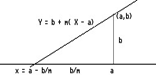where $f$ is a differentiable function. The equation's form is not very restrictive since an equation of the more general form $P(x) = Q(x)$ has the same solutions as the equation $f (x) = P(x) - Q(x) = 0$.

The key to the method is the simplicity of solving for the X-intercept of a non-horizontal line.
For example, find the X intercept of the line with slope of $2$ passing through the point $(1,-1)$. More generally, find the solution to the equation $L(x) = 0$ when $L$ is a non-constant linear function with slope $m$ and $L(a) = b$. Recall that if a line passes through the point $(a,b)$ and has slope $m$ (not equal to $0$) then the line has equation $Y = b + m( x - a)$. By setting $Y = 0$ we find that the X-intercept is $x = a - b/m$. (See Figure 1)
So, the line passing through the point $(1,-1)$ with a slope of $2$ has its X-intercept  given by $x = 1 - (-1)/2 = 3/2$.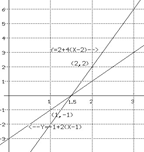Or if the line passes through the point $(2,2)$ with a slope of $4$ then its X-intercept will be given (coincidently) by $x = 2 - (2)/4= 3/2$. (See Figure 2.)

For a non linear example, we estimate $\sqrt 2$ with Newton's method. Of course there are more exciting examples, but we'll stay with a number which is familiar as a starter.
We begin by recognizing that we are trying to solve the equation $x^2 = 2$. which can be transformed to the equation $x^2 - 2 = 0$. To use Newton's method we examine the
function $f (x) = x^2 - 2$. [These are the same initial considerations found in the use of the Figure 2 for the bisection method.]

Now here's the first application of Newton's method to finding an estimate of  $\sqrt 2$ .As noted above we consider $f (x) = x^2 - 2$, and since we will use the derivative of $f$, interpreted as the slope of the tangent line at x, we note also that $f '(x) = 2x$.

Step 1:  By some technique get a first estimate of the solution. Call it $x_0$.  $x_0$ is sometimes called the "seed" for the method.
For the $\sqrt 2$  we will use $x_0 = 1$.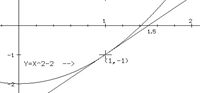[Notice that $f (1) = -1$ and $f (2) = 2$, so by an application of the intermediate value  theorem  we see that there is a solution to the equation $f (x) = 0$  between $1$ and $2$. Since $f (1)$ is closer to $0$ than $f (2)$ we'll start with the choice of $x_0 =1$.]
For the notation we will use in the general case later, let $y_0 = f (x_0) = f (1) = -1$.
Now compute the derivative at $x_0$ .
We again introduce notation, letting $y'_0 = f'(x_0) = 2 x_0 = 2(1) = 2$. So we have that the line tangent to the graph of $f(x)$ at the point $(1,-1)$ has a slope of $2$.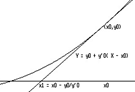Step  2:  Here is the key estimation idea, use the tangent line at $(x_0 ,y_0) = (1,-1)$  with its slope of $y'_0 = 2$ to approximate the function $f$.  So to estimate where $f (x) = 0$,  find the X-intercept of the tangent line, which we  call $x_1$. By our previous work about the intercept of a line, we find the X-intercept is $x_1 = 1- (-1)/2 = 3/2$ .  See Figure 3. [Thus $x_1 = x_0 - y_0 / y'_0$. See Figure 4.]

This number, $x_1$, is the next estimate for the solution to the equation.
Step 3:Repeat step 1 now using $x_1$ instead of $x_0$  for the estimate of the solution. I.e., compute  $y_1 = f (x_1) = f (3/2) = (9/4)-2 = 1/4$ and $y '_1= f '(x_1) = 2 x_1 =3$.

Step 4: Repeat step 2, now using the tangent line at $(x_1,y_1) = (3/2,1/4)$ with slope $y'_1 = 3$ to approximate $f$ and obtain the next estimate of the solution from the X-intercept of this tangent line.    Figure III.4
Thus $x_2 = x_1 - y_1 / y'_1 = (3/2) - (1/4)/3 = 17/12 \approx 1.417$.
Step 5: Continue as in Steps 3 and 4 until the estimate is "good enough" (or it becomes apparent that the method is not working).
So for example, $x_3 = x_2 - y_2/y '_2$, where   $y_2 = f (x_2)$ and $y'_2= f '(x_2); x_4 = x_3 - y_3/y'_3$,  and in general

$x_{n+1} = x_n - y_n/y'_n$.
Rather than continue further with these calculations, here is a formula which tells how to find the next $(n+1)st$ estimate for $\sqrt 2$  in general based on the previous (n)th estimate: $x_{n+1} = x_n - \frac {x_n^2 - 2}{2x_n} \\ = \frac {x_n^2 +2}{2x_n} = \frac {x_n}2 +\frac 1 {x_n}$.

Using this formula and the fact that $x_2 =17/12$ we make the next (3rd) estimate of  $\sqrt 2$
$x_3 = x_{2+1} = \frac {x_2}2 +\frac 1 {x_2}= \frac {17/12}2 + 12/17 = \frac {577}{24 \cdot 17} =\frac {577}{408} \approx 1.414257$.

Now examine Table 1 - an active spreadsheet created using GeoGebra.
 Table 1

Notice how close this estimate is compared to the value for the $\sqrt 2$
[1.41421356237 is the estimate given by the HP48G.]

 Summary of Newton's Method Step 1: Make a first estimate of the solution. Call it $x_0$.  $x_0$ is sometimes called the "seed" for the method. Let $y_0 = f (x_0)$. Compute the derivative at $x_0$, $y'_0 = f'(x_0)$. Step  2: Use the tangent line at $(x_0,y_0)$  with its slope of $y'_0$ to approximate the function $f$. Find the X-intercept of the tangent line, which we call $x_1$.  This number is the next estimate for the solution to the equation. Thus $x_1 = x_0 - y_0 / y'_0$.  See Figure III.4. Step 3: Repeat step 1 now using $x_1$ instead of $x_0$  for the estimate of the solution.Step 4: Repeat step 2, now using the tangent line at $(x_1,y_1)$ with slope $y'_1 = f (x_1)$ to approximate $f$ and obtain the next estimate of the solution from the X-intercept of this tangent line. Thus  $x_2 = x_1 - y_1 / y'_1$ . Step 5: Continue as in Steps 3 and 4 until the estimate is "good enough" (or it becomes apparent that the method is not working).  In a single equation, the key formula for finding $x_{n+1}$  using $x_n$  is $x_{n+1} = x_n - y_n/y'_n = x_n - f (x_n)/f '(x_n)$

Here's another example, this time finding a cube root:
Example III.A.?? Use Newton's Method to estimate $\sqrt 5$. .
Solution:  To find $\sqrt 5$ we recognize that this number is characterized by being a solution to the equation $x^3 =5$. So we consider the function $f (x) = x^3 - 5$ and use Newton's method to look for solutions to the equation $f (x)= x^3- 5 = 0$.

As Newton's method will use the derivative of $f$  repeatedly, we note that $f '(x)= 3x^2$.
Since $f (1) = -4$ and $f (2) = 3$ we can apply the Intermediate Value Theorem to conclude that the number we seek is between $1$ and $2$. We'll start our estimation with $2$ as the seed since $3$ is closer to $0$ than $-4$ .
 Table 2

Step 1: Let $x_0 = 2$. So $y_0 = f (x_0) = 3$ and $y'_0 = f '(x_0) = 3(4) = 12$.
Step 2: $x_1 = x_0 - y_0/y '_0$,  so $x_1 = 2 - 3/12 = 7/4$.
Step 3: $y_1 = f (x_1) = (7/4)^3 - 5 = .359375$ and $y'_2 = 3(7/4)^2 = 9.1875$.
Step 4: $x_ 2 = x_1 - y_1/ y '_1$ , so  $x_2 = 1.75 - (.359375/9.1875) \approx 1.71088$.
To see how the method continues see Table 2 created with GeoGebra

Note on a Newton's Function and Iteration: Since $x_{n+1} = x_n - y_n/y'_n = x_n - f (x_n)/f '(x_n)$, we can define a function $N(x) = x - f (x )/f '(x)$. Thus $x_{n+1} = N( x_n )$. Once this function is determined, the repeated application of Newton's method corresponds precisely to the repeated application of the function $N$ to the result of applying the function $N$. Using Newton's function $N$ on a spreadsheet or a calculator to estimate the root or zero of a function consolidates the computations and allows a simpler presentation.

For finding $\sqrt 2$ , Newton's function is $N(x) = x- \frac{x^2-2}{2x} = \frac x 2 +\frac 1 x$.
For finding  $\sqrt5$, Newton's function is $N(x) = x- \frac{x^3-5}{3x^2} = \frac 23 x +\frac 5 {3x^2}$.

Table 3 gives a table created with GeoGebra that results from applying Newton's functions repeatedly to the seed $x_0=1$ for estimating $\sqrt 2$ and $\sqrt 5$.
 Table 3

Interpretations: We have already seen the graphical interpretation of Newton's method using the tangent line to estimate when a function will cross the X-axis. The  motion interpretation of the method is explored more fully in the exercises at the end of this section. We will explore the economic interpretation in terms of marginal profits briefly.

Economic Interpretation: When a business starts producing some commodity, the initial levels of costs and revenues may be unbalanced resulting in a negative profit (otherwise described by investors as a loss). The main issue for management is what level of production (and sales) will lead to achieving a desired level of profit.
For example, if we let $P( x)$ denote the profit in dollars for producing $x$ kilograms, the issue would be to find an estimate of the appropriate $x$ so that the profit would be $\$1000$. We can restate this as trying to estimate a solution for the equation$P( x) = 1000$, or$P(x)-1000 = 0$. The management might plan to do this is stages, rather than believe that their first estimate would be accurate. Suppose that initially the business produces 40 kilograms and makes a profit of$\$700$. If we suppose further that the profit from producing another kilogram at this level of production is $\$30$, we can apply Newton's method. What would the method mean in terms of this profit interpretation? Let$f (x) = P(x) - 1000$, then$f (x)$measures the difference between the actual profit and the profit goal. In the example we have set up so far,$f(40)= 700 -1000 = -300.$The management's objective is to estimate$x$so that$f(x)=0$, that is, to produce enough so there is no difference between the actual profit and the goal. To determine an estimate for the next production level with Newton's method, we use both the initial production,$x_0 = 40$and the marginal profit rate,$P'(40)=30 = f '(40)$. The management will need to increase the profit by$\$300$ to meet their goal of a zero difference. To achieve this much profit at the given marginal rate, they should produce an additional $300/30 = 10$ kilograms.
So the management should set the next production level at
$x_1 = x_0 - f (x_0)/f '(x_0) = 40 - (-300)/10 = 50$ kilograms. At this stage the management will wait to see how this level of production effects the profit and reaching the desired level of profit. If the difference is not close enough to 0, the management will determine the marginal profit at that level of production and adjust the production level again to reduce the difference based on the rate of marginal profit (using Newton's method).

Probability Interpretation: Recall that the median of a continuous random variable X is that number M where the probability that the value of X is less than or equal to M is 1/2 .
In terms of the distribution function $F$ for X, we are trying to solve the median equation $F(M) = 1/2$ or $F(M) - 1/2 = 0$.
Because $F$ is a continuous distribution function we know already that the Intermediate Value Theorem applies to $F$ and there is a median.
We can choose any number $M_0$ for a guess of what the median might be and find $F(M_0)$.

Now Newton's method finds the next estimate, $M_1$, using the formula
$M_1 = M_0 - \frac{F(M_0) - \frac 12}{F'(M_0)}$.
The interpretation of the method will involve interpreting $F '$, the probability density of the random variable X.
Interpretation: The magnitude of the number $F(M_0) - 1/2$ measures the probability that X is between $M_0$ and $M$. When this difference is close to 0 then $M_0$ is a good estimate of the median. Notice that the average probability density for X in the interval between $M_0$ and $M$ is precisely the quotient $(F(M_0) - 1/2)/(M_0 -M)$.
To find the difference between $M_0$ and $M$ we would divide the probability that X is between $M_0$ and $M$ by the associated average probability density.
For a Newton's method estimation of M, we  approximate this average probability density (which we don't know) with the point probability density of X at $M_0$, that is $F'(M_0)$. Thus to estimate the difference between$M_0$ and $M$ we would divide the probability that X is between $M_0$ and $M$ by the associated probability density of X at $M_0$.
The arithmetic of the situation  therefore makes it sensible to make the next estimate $M_1$ as the initial estimate $M_0$ less the estimate of the difference $M_0 - M$ given by
$(F(M_0) - 1/2)/F'(M_0)$.
So we have arrived at Newton's method again
$M_1 = M_0 - \frac{F(M_0) - \frac 12}{F'(M_0)}$.

To be more specific we can think of the random variable that measures the distance from the center in the darts example on a dart board of radius 4 where
$A^2/16$ for A in the interval $[0,4]$,
$F(A)= 0$ when $A < 0$, and
1 when   $A>4$.
In this case we can solve the median equation $\frac{M^2}{16} - \frac 12 = 0$ and find  $M = 2 \sqrt 2 \approx 2.828$ . Since we are trying to understand Newton's method here, we'll put that information aside and try to approximate this solution.
Since $F(2) = 1/4$ and $F(3)=9/16$ we can see that $M$ is between $2$ and $3$. Choose $M_0 =3$ for the seed because $9/16 - 1/2 = 1/16$ is fairly close to $0$. Therefore 3 is not a bad estimate for the median.
To improve the estimate of the median, we approximate the difference between $3$ and the actual median. Use $F '(M_0) = F '(3) = 3/8$  the point probability density at $3$ to estimate the average probability density for the interval between $3$ and $M$.
The difference between $3$ and $M$ is thus estimated by the quotient of the probability that the random variable is between $3$ and $M$ by the point probability density at 3, that is, $1/16$ divided by $3/8$ or $(1/16)/(3/8)=1/6$.
Since it is clear that $3$ is larger than the median, the next estimate should be reduced by $1/6$, so the revised estimate of the median for this random variable is $3 -1/6 = 2 \frac 56$.
This is precisely what we obtain from Newton's method, namely:
$M_1 = M_0 - \frac{F(M_0) - \frac 12}{F'(M_0)} = 3 - \frac{9/16 -1/2} {3/8} = 3 - \frac 16 = 2 \frac 56$.

 k $x_k$ 0 0.5 1 -0.750000000000000 2 0.291666666666667 3 -1.56845238095238 4 -0.465440611728562 5 0.841530602630985 6 -0.173390155939164 7 2.79697497406861 8 1.21972292723823 9 0.199932299516144
Question 1: Does Newton's method always lead to better estimates for a solution of $f(x )= 0$?
Response: Of hand, without any other tools or information about the function f , Newton's method can be applied repeatedly without improving the quality of the estimate.

In the first place, there may not be any solution to the equation.
For example, the equation $x^2 + 1 = 0$ has no real number solutions. [It does have two solutions, but they are the imaginary  numbers i and -i.] So if you apply Newton's method to this problem starting with a seed of $x_0=1/2$ you will obtain a list of numbers, none of which will be "closer" to the solution! See Table 4.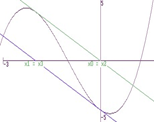Another way that Newton's method can fail to improve the quality of its estimates of a solution (assuming there is a solution) is in a case when an estimate repeats. Figure III.5 visualizes this potential failure with a pair of tangent lines forming a cycle of estimates.

Finally another unfortunate aspect of Newton's Method is that the algorithm in fact may lead to a worse estimate of the
solution. The sources of this kind of extreme error in the method are mainly from situations where the tangent line intersects the X-axis relatively far from the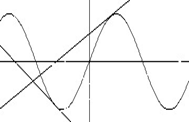actual solution and where the slope of the tangent line is close to or equal to zero. For examples of these difficulties see Figure III.6 and exercise 5.

Question 2. Is there a way to tell how accurate the estimate from Newton's method is?
Response:
Unlike the bisection method discussed in Chapter I.I, Newton's method does not have a built in calculation to help determine the accuracy of the estimate for the solution of $f(x)=0$.
Fortunately, we can still use the Intermediate Value Theorem for a continuous function to check on the quality of the estimate.  Thus, for example if $f (x_n)>0$ and $f (x_{n} + \epsilon)<0$ where $\epsilon$ is some nonzero number, then at least one solution is between $x_n$ and $x_{n} + \epsilon$.
So if we believe that $x_n$ is  within $0.01$ of the actual solution we could evaluate $f (x_n), f (x_n+.01)$, and $f (x_n - .01)$ to see if there is a change in sign of these values- which would guarantee the accuracy by the Intermediate Value Theorem.

Question 3. Is there a way to tell how many times Newton's method will need to be applied to obtain an estimate of a desired accuracy?
Response: The short and not too happy answer to this question is" no!" Unlike the bisection method, the predictability of Newton's method is not good. Because a function can vary greatly before crossing a particular value, the derivative and the estimates that it will generate through Newton's method will be unpredictable. Fortunately, in most textbook applications the method will be quite rapid in finding a solution in comparison to the bisection method. This leads to a trade off between the frequently apparent great efficiency of Newton's method and the certain effectiveness and relative efficiency of the bisection method.
Having both the bisection and Newton's methods at hand is a good way to approach a problem, deciding as you proceed which method is best depending on how the methods seem to be working. [Many technology based equation solvers use both these methods in some hybrid fashion.]

Exercises III.A.2: In each of the following problems, use Newton's method to estimate the solution of the given equations by finding  $x_1, x_2, x_3$, and $x_4$.
Warning: You may need to adjust the initial $x_0$ in some of these problems to proceed to a decent estimate of the solution. In each problem discuss briefly the quality of $x_4$ as an approximate solution.
1.    $x^2 - 5 = 0$. (Start with $x_0 = 2$.)
2.    $x^5 + 2 = 0$. (Start with $x_0 = -1$.)
3.    $x^3 - 7 = 0$. (Start with $x_0 = 2$.)
4.    $x^3 - 2x - 3 = 0$.  (Start with $x_0 = 2$.)
5.    a.  $x^3 + 3x^2 - 2x - 4 = 0$.  (Start with $x_0 = 0$.)
b.  $x^3 + 3x^2 - x + 1 = 0$.   (Start with $x_0 = 1$.)
6. $2x^3 - 4x + 1 = 0$.  (Start with $x_0 = 1$.)
7. $x^3 = 3x + 1$ .  (Start with $x_0 = 1$.)
8.    a.  $\sin(x) = .5$ .          b.  $\tan(x) = 1$.
9.    a.  $\sin^2(x) = .5$  .       b.  $\tan(x) = x + 1$.
10.    Use Newton's method to approximate correctly $\sqrt {56}$ to the nearest hundredth. Discuss briefly the reasoning for the accuracy of your result.
11.    Use a spreadsheet and  Newton's method to approximate the solution to problem 10 correctly to the nearest millionth. Compare the result with the result given by a hand-held calculator.
12.   Use a spreadsheet and  Newton's method to approximate $\sqrt {56}$ correctly to the nearest millionth. Compare the result with the result given by a hand-held calculator.
13.    Use Newton's method to justify the following formula for approximating the square root of 3 assuming $x_0 = 1$:
$x_{n+1} = (x_n^2 + 3)/2x_n$ . Use this method to estimate  $\sqrt 3$ correct to 1000th's.
13. a.Use Newton's method to generalize problem 13, giving a method for estimating with any required accuracy the square root of any positive real number $a > 0$ by iterations.
b. Use Newton's method  to give a method for estimating with any required accuracy the cube root of any real number $a$ by iterations.
14. Write a short discussion on how you would respond to the following situations that can occur in using Newton's Method to solve $f(x) = 0$. Briefly explain why your response might be helpful to resolving the situation.
In each case assume that f  is a continuous function, $f (-1) = -10, f (1) = 10$ and $x_0 = 1$.
a. Using Newton's Method you find $x_1 = x_3 = -1$ and $x_0 = x_2$.
b.    Using Newton's Method you find $x_1 = x_3 = 0$and $x_0 = x_2$.
c.    Using Newton's Method you find $x_1 = -2$ and $x_2 = 3$.
d.    Using Newton's Method you find  $f '(1) = 0$.
e.    Using Newton's Method you find  $x_1= 0$ and $f '(0) = 0$.
15.    The text gave a tangent line interpretation for Newton's method  for  the approximate solution of the equation $f (x) = 0$.  Consider $f (x)$ as the position of a moving object as in a mapping diagram.  The question of solving $f (x) = 0$ can be interpreted as asking when the moving object is at position $0$. If the object is at position $f (a)$ at time $a$ and is traveling at constant velocity $f '(a)$, explain in terms of this interpretation why $a - f(a)/f '(a)$ is an appropriate estimate for the solution of this problem.
16.    Project: An interpolation method for estimating the solution to $f (x)= 0$. Suppose f is a continuous function with $f(a)>0$ and $f(b)<0$.  By the Intermediate Value Theorem $f(x) = 0$ has solution between $a$ and $b$. Find the X-intercept of the line connecting $(a,f(a))$ to $(b,f(b))$.
Using this X-intercept as a new estimate of the solution to $f (x)=0$. Develop an iterative method based on this idea to estimate the solution  to $f (x) = 0$.  Use your method to estimate solutions for problems 1 through 3. Compare the effectiveness of this method with the both Newton's method and the bisection method. What advantages does this method have in comparison with Newton's Method? with the bisection method?
17.   Discuss the following GeoGebra application that illustrates Newton's method for finding the roots of $f(x) = (x-1)(x+1.5)(x+2)$ with a speadsheet, a graph and a mapping diagram. How are the three tools similar? how do they differ? How does the seed $x_0$ effect which root is found.